Next: Radio Wave Propagation Through Up: Waves in Cold Plasmas Previous: Pulse Propagation

# Ray Tracing

Let us now generalize the preceding analysis so that we can deal with pulse propagation though a three-dimensional magnetized plasma.

A general wave problem can be written as a set of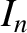coupled, linear, homogeneous, first-order, partial-differential equations, which take the form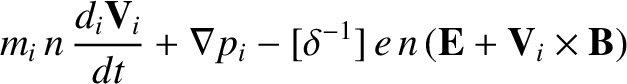(639)

The vector-field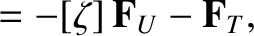hascomponents (e.g.,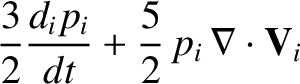might consist of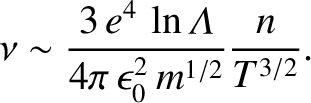,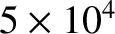,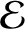, and) characterizing some small disturbance, and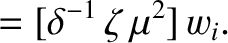is an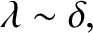matrix characterizing the undisturbed plasma.

The lowest order WKB approximation is premised on the assumption thatdepends so weakly on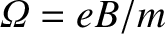and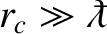that all of the spatial and temporal dependence of the components of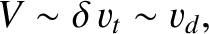is specified by a common factor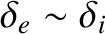. Thus, Eq. (639) reduces to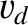(640)

where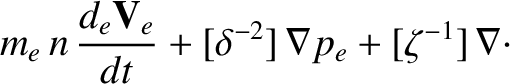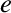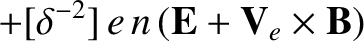(641)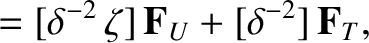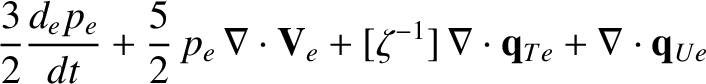(642)

In general, Eq. (640) has many solutions, corresponding to the many different types and polarizations of wave which can propagate through the plasma in question, all of which satisfy the dispersion relation(643)

where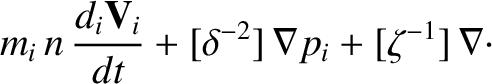. As is easily demonstrated (see Sect. 4.11), the WKB approximation is valid provided that the characteristic variation length-scale and variation time-scale of the plasma are much longer than the wave-length,, and the period,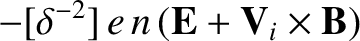, respectively, of the wave in question.

Let us concentrate on one particular solution of Eq. (640) (e.g., on one particular type of plasma wave). For this solution, the dispersion relation (643) yields(644)

i.e., the dispersion relation yields a unique frequency for a wave of a given wave-vector,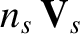, located at a given point,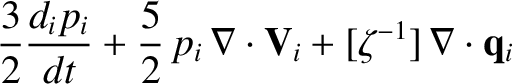, in space and time. There is also a uniqueassociated with this frequency, which is obtained from Eq. (640). To lowest order, we can neglect the variation ofwithand. A general pulse solution is written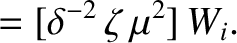(645)

where (locally)(646)

and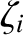is a function which specifies the initial structure of the pulse in-space.

The integral (645) averages to zero, except at a point of stationary phase, where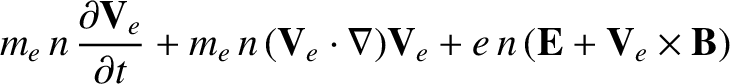(see Sect. 4.16). Here,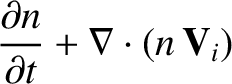is the-space gradient operator. It follows that the (instantaneous) trajectory of the pulse matches that of a point of stationary phase: i.e.,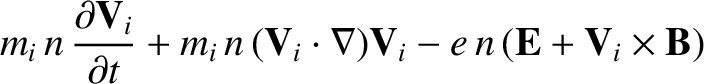(647)

where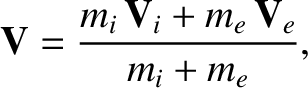(648)

is the group velocity. Thus, the instantaneous velocity of a pulse is always equal to the local group velocity.

Let us now determine how the wave-vector,, and frequency,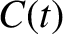, of a pulse evolve as the pulse propagates through the plasma. We start from the cross-differentiation rules [see Eqs. (641)-(642)]: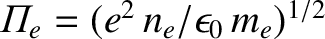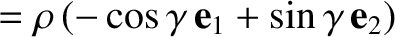(649)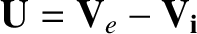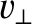(650)

Equations (644) and (649)-(650) yield (making use of the Einstein summation convention)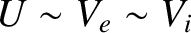(651)

or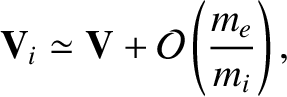(652)

In other words, the variation of, as seen in a frame co-moving with the pulse, is determined by the spatial gradients in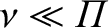.

Partial differentiation of Eq. (644) with respect togives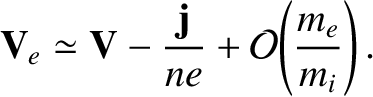(653)

which can be written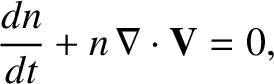(654)

In other words, the variation of, as seen in a frame co-moving with the pulse, is determined by the time variation of.

According to the above analysis, the evolution of a pulse propagating though a spatially and temporally non-uniform plasma can be determined by solving the ray equations: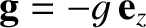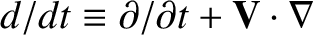(655)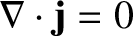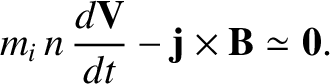(656)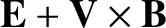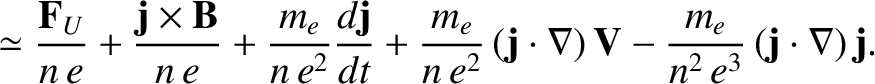(657)

The above equations are conveniently rewritten in terms of the dispersion relation (643):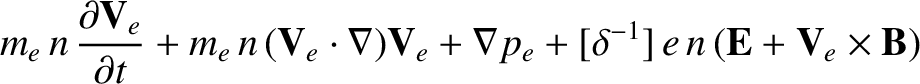(658)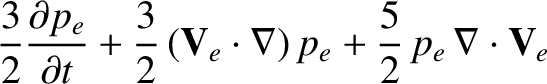(659)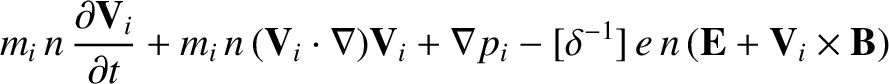(660)

Note, finally, that the variation in the amplitude of the pulse, as it propagates through though the plasma, can only be determined by expanding the WKB solutions to higher order (see Sect. 4.11).Next: Radio Wave Propagation Through Up: Waves in Cold Plasmas Previous: Pulse Propagation
Richard Fitzpatrick 2011-03-31